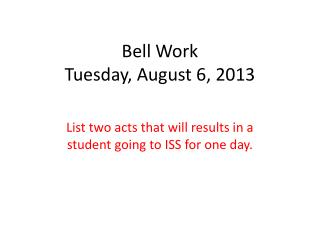Download PresentationBell Work Tuesday, August 6, 2013

# Bell Work Tuesday, August 6, 2013

Download Presentation## Bell Work Tuesday, August 6, 2013

- - - - - - - - - - - - - - - - - - - - - - - - - - - E N D - - - - - - - - - - - - - - - - - - - - - - - - - - -
##### Presentation Transcript

1. Bell WorkTuesday, August 6, 2013 List two acts that will results in a student going to ISS for one day.

2. Standard • MM4A9. Students will use sequences and series. • a. Use and find recursive and explicit formulas for the terms of sequences.

3. I can statement • I can review arithmetic sequence and geometric sequence.

4. Fibonacci Sequences • http://www.youtube.com/watch?v=dr20wqKYYtQ

5. Arithmetic Sequences Every day a radio station asks a question for a prize of \$150. If the 5th caller does not answer correctly, the prize money increased by \$150 each day until someone correctly answers their question.

6. Arithmetic Sequences Make a list of the prize amounts for a week (Mon - Fri) if the contest starts on Monday and no one answers correctly all week.

7. Arithmetic Sequences • Monday : \$150 • Tuesday: \$300 • Wednesday: \$450 • Thursday: \$600 • Friday: \$750

8. Arithmetic Sequences • These prize amounts form a sequence, more specifically each amount is a term in an arithmetic sequence. To find the next term we just add \$150.

9. Definitions • Sequence: a list of numbers in a specific order. • Term: each number in a sequence

10. Definitions • Arithmetic Sequence: a sequence in which each term after the first term is found by adding a constant, called the common difference (d), to the previous term.

11. Explanations • 150, 300, 450, 600, 750… • The first term of our sequence is 150, we denote the first term as a1. • What is a2? • a2 : 300 (a2 represents the 2nd term in our sequence)

12. Explanations • a3 = ? a4 = ? a5 = ? • a3 : 450 a4 : 600 a5 : 750 • an represents a general term (nth term) where n can be any number.

13. Explanations • Sequences can continue forever. We can calculate as many terms as we want as long as we know the common difference in the sequence.

14. Explanations • Find the next three terms in the sequence: 2, 5, 8, 11, 14, __, __, __ • 2, 5, 8, 11, 14, 17, 20, 23 • The common difference is? • 3!!!

15. Explanations • To find the common difference (d), just subtract any term from the term that follows it. • FYI: Common differences can be negative.

16. Geometric Sequences

17. GeometricSequence • What if your pay check started at \$100 a week and doubled every week. What would your salary be after four weeks?

18. GeometricSequence • Starting \$100. • After one week - \$200 • After two weeks - \$400 • After three weeks - \$800 • After four weeks - \$1600. • These values form a geometric sequence.

19. Geometric Sequence • Geometric Sequence: a sequence in which each term after the first is found by multiplying the previous term by a constant value called the common ratio.

20. Geometric Sequence • Find the first five terms of the geometric sequence with a1 = -3 and common ratio (r) of 5. • -3, -15, -75, -375, -1875

21. Geometric Sequence • Find the common ratio of the sequence 2, -4, 8, -16, 32, … • To find the common ratio, divide any term by the previous term. • 8 ÷ -4 = -2 • r = -2

22. Vocabulary • arithmetic sequence • geometric sequence • common difference • common ratio

23. Create a sequence for your elbow partner • Have your elbow partner determine whether its an arithmetic sequence, geometric sequence, or neither.

24. Closing • See nature by numbers video:http://www.youtube.com/watch?v=kkGeOWYOFoA • Homework Assignment #1 – You saw the video, Nature by Numbers. Submit a picture of something from nature and point out one or several geometric shapes similarly to what you saw in the video. Due: Wednesday in class.